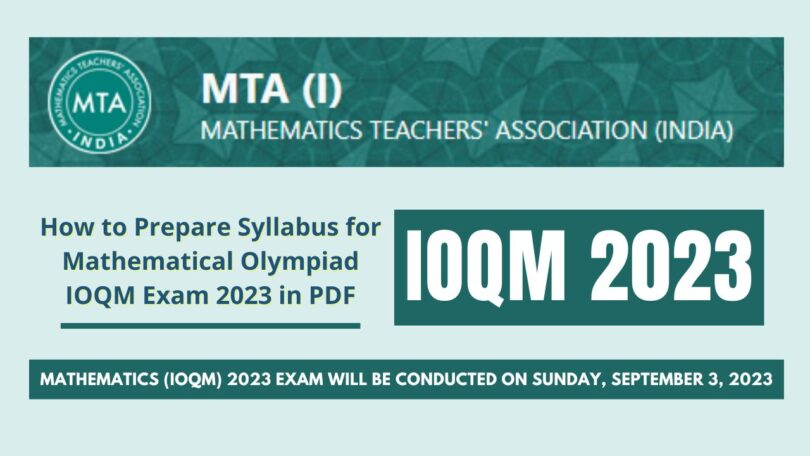# How to Prepare Syllabus for Mathematical Olympiad IOQM Exam 2023 in PDFWritten by

Are you searching for – How to Prepare Syllabus for Mathematical Olympiad IOQM Exam 2023 in PDF

Then you are at Right Place.

The Complete and Official Information of How to Prepare Syllabus for Mathematical Olympiad IOQM Exam 2023 in PDF

## How to Prepare Syllabus for Mathematical Olympiad IOQM Exam 2023 in PDF

We are giving official information regarding upcoming examination of the Mathematics – IOQM 2023

### Syllabus for IOQM – 2023 (Mathematical Olympiad)

The syllabus for Mathematical Olympiad (regional, national and international) is pre-degree college mathematics.

The areas covered are arithmetic of integers, geometry, quadratic equations and expressions, trigonometry, co-ordinate geometry, the system of linear equations, permutations and combination, factorization of polynomial, inequalities, elementary combinatorics, probability theory and number theory, finite series, and complex numbers, and elementary graph theory.

The syllabus does not include calculus and statistics. The major areas from which problems are given are algebra, combinatorics, geometry, and number theory.

The syllabus is in a sense spread over Class XI to Class XII levels, but the problems under each topic involve a high level of difficulty and sophistication.

The difficulty level increases from RMO to INMO to IMO.